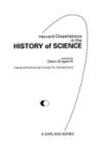## Pitzer Faculty Books#### Title

The Calculus as Algebra: J. L. Lagrange, 1736-1813

#### Description

In The Calculus as Algebra: J.-L. Lagrange, 1736–1813, Grabiner shows what Lagrange’s mathematical practice was like, in order to understand the genesis of the rigorous analysis of Cauchy, Bolzano, and Weierstrass. For Lagrange, the calculus was not about rates of change or ratios of differentials, or even about limits as then understood. Lagrange thought that the calculus should be reduced to “the algebraic analysis of finite quantities.” This sounds as though he was about to introduce deltas and epsilons. But instead he believed that there was an algebra of infinite series, and that every function had a power-series expansion except perhaps at finitely many isolated points. Lagrange defined the derivative as the coefficient of the linear term in the function’s power-series expansion. Why he thought this was justified tells us both about his philosophy of mathematics and about the way many mathematicians practiced their subject in the eighteenth century.

0824074483

1990

#### Publisher

Garland Publishing

New York

#### Keywords

mathematics, history

#### Disciplines

Analysis | History | MathematicsCOinS